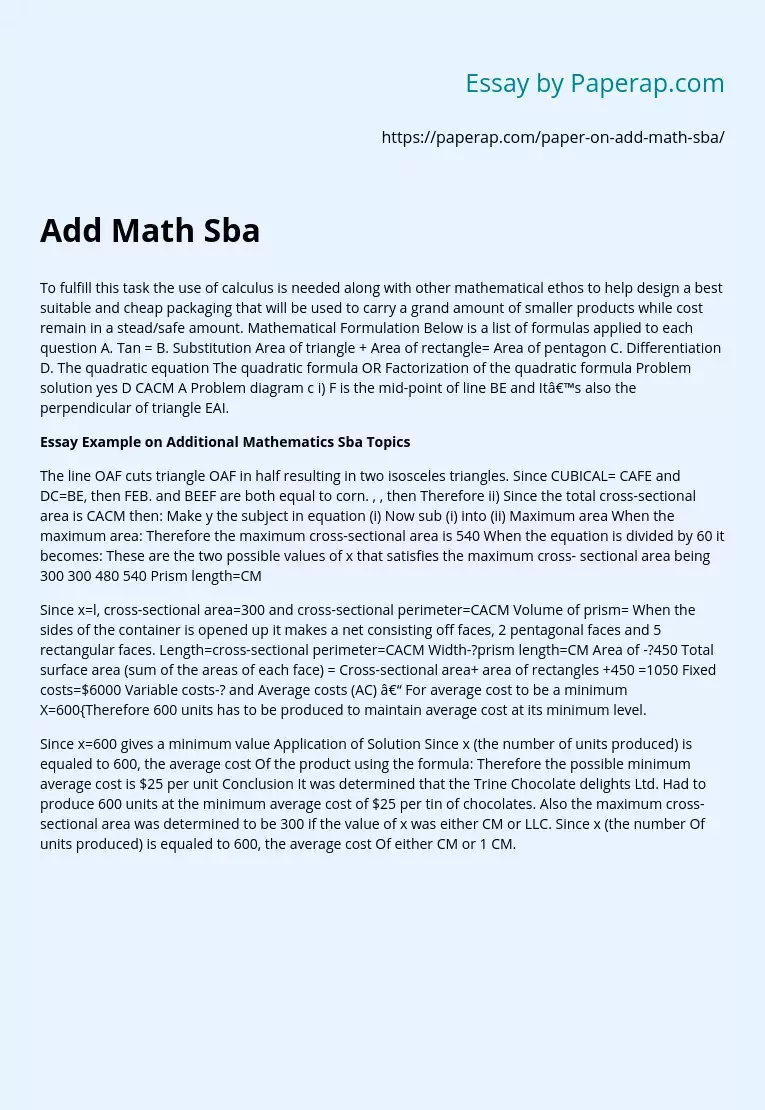# Additional Mathematics Sba Topics

To fulfill this task the use of calculus is needed along with other mathematical ethos to help design a best suitable and cheap packaging that will be used to carry a grand amount of smaller products while cost remain in a stead/safe amount. Mathematical Formulation Below is a list of formulas applied to each question A. Tan = B. Substitution Area of triangle + Area of rectangle= Area of pentagon C. Differentiation D. The quadratic equation The quadratic formula OR Factorization of the quadratic formula Problem solution yes D CACM A Problem diagram c i) F is the mid-point of line BE and It’s also the perpendicular of triangle EAI.

## Essay Example on Additional Mathematics Sba Topics

The line OAF cuts triangle OAF in half resulting in two isosceles triangles. Since CUBICAL= CAFE and DC=BE, then FEB. and BEEF are both equal to corn. , , then Therefore ii) Since the total cross-sectional area is CACM then: Make y the subject in equation (i) Now sub (i) into (ii) Maximum area When the maximum area: Therefore the maximum cross-sectional area is 540 When the equation is divided by 60 it becomes: These are the two possible values of x that satisfies the maximum cross- sectional area being 300 300 480 540 Prism length=CM

Since x=l, cross-sectional area=300 and cross-sectional perimeter=CACM Volume of prism= When the sides of the container is opened up it makes a net consisting off faces, 2 pentagonal faces and 5 rectangular faces.

Length=cross-sectional perimeter=CACM Width-?prism length=CM Area of -?450 Total surface area (sum of the areas of each face) = Cross-sectional area+ area of rectangles +450 =1050 Fixed costs=\$6000 Variable costs-? and Average costs (AC) – For average cost to be a minimum X=600{Therefore 600 units has to be produced to maintain average cost at its minimum level.

Get quality help nowSweet VVerified

Proficient in: Geometry4.9 (984)

“ Ok, let me say I’m extremely satisfy with the result while it was a last minute thing. I really enjoy the effort put in. ”+84 relevant experts are online

Since x=600 gives a minimum value Application of Solution Since x (the number of units produced) is equaled to 600, the average cost Of the product using the formula: Therefore the possible minimum average cost is \$25 per unit Conclusion It was determined that the Trine Chocolate delights Ltd. Had to produce 600 units at the minimum average cost of \$25 per tin of chocolates. Also the maximum cross-sectional area was determined to be 300 if the value of x was either CM or LLC. Since x (the number Of units produced) is equaled to 600, the average cost Of either CM or 1 CM.